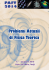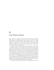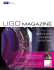# Gravitational Frequency Shift for Weyl

## Transcription

Gravitational Frequency Shift for Weyl
```Inveltigación
Revista Mexicana de Física 33 No. 2(1987) 189-199
General Equations of Motion in the Solar System:
Gravitational Frequency Shift for Weyl-affine
Theories of Gravity
A. Sarmiento
Instituto
G.
Apdo. Posta/ 70-26~, O~51O México, D.F.
de "féxico,
and
A.A. Coley
Dept. 01 Mathematics, Statistics and Gomputing Science,
Da/housie University. Ha/ilax, Nova Scotia. Ganada B9H 4H8
(recibido el 21 de noviembre de 1986; aceptado el 24 de abril de 1987)
Abstract. A formalism in which a class of non-metric theories of
gravity can be analyzed, is extended so that the nature of possible
eonstraints arising from the outcome oC a planned solar probe experiment can be investigated. The modelling of the planned solar
mission allows the gravitational frequency shift effect predicted by
these theories to be ealculated (up to third order in the Newtonian
gravitational potential). The comparison with general relativity
theory predictions place s strong limits on the possible non-metric
nature of spacetime within the solar system.
Resumen. Se extiende un formalismo para el análisis de una
clase de teorías no-métricas con el objetivo de investigar la naturaleza de las posibles restricciones que puedan resultar de un experimento solar planeado. Mediante un modelo de la misi6n solar,
se calcula el efecto del corrimiento gravitacional en frecuencia que
predicen estas teorías (a tercer orden en el potencial gravitacional
newtoniano). Al comparar los cá.lculos con las predicciones de la
teoría general de la relatividad, se obtienen severos límites sobre
la posible naturaleza no-métrica del espacio-tiempo en el sistema
solar.
PACS: 04.20.Me; 04.50.+h; 04.80.+z; 95.10.Eq; 95.30.Sf
184
A. Sarmiento G. and A.A. Coley
1. Introduction
In a recent article [11, hereinafter referred to as paper I, a formalism for the analysis of a class of non-metric theories of gravity was
presented. The formalism, accurate to third order in the Newtonian
gravitational potential U, was used to compute the gravitational
frequency shift effect predicted by Weyl-affine theories of gravity
(WATG's) in a spherically syrnmetric and static (SSS) gravitational
field for a hypotetical experimental situation in which both an artificial satellite and the Earth followed circular coplanar orbits around
the Sun. Using available experimental limits it was then shown that
(WATG's) had to be ."metric" to first order in U.
It is the aim of this article to investigate in greater depth the
nature of possible constraints on WATG 's arising from the out come
of a planned solar probe experiment in which both the satellite and
the Earth follow general (non-circular) orbits. For the sake of brevity
we shall often refer to equations in paper I (e.g., Eq. (1.2.1) refers to
equation (2.1) in paper I). The notation is identical to that used in
paper 1.
A brief review of the class of non-metric theories of gravity called
Weyl-affine theories is presented in section 2.
A formalism is then designed and applied, in section 3, to the
modelling of a planned solar miss ion in which the Earth and a satellite move around the Sun following the coplanar orbits determined
by the gravitational solar field.
The calculations of the gravitational frequency shift up to third
order in U are then carried out both for Weyl-affine theories in
which >. is strictly non constant and for general relativity theory
(an example of a metric theory of gravity in which >. = 1). Finally,
in section 4 the results are preseated and discussed and the level at
which the possible non-metric nature of spacetime would affect the
outcome of the planned experiment is analyzed.
------------_.-_.
General Equations o/ Motion in the Solar ...
185
2. A Class of Non-Metric Theories of Gravity and their Description in a Spherically Symmetric and Static Gravitational Field
As was discussed in paper 1, there exists a class of non-metric
theories of gravity, called Weyl-affine theories of gravity (WATG's),
which satisfy all the current conditions of viability. Essentially, a
WATG is a geometric theory of gravity (i.e., spacetime is characterized by a four-dimensional, Hausdorlf, dilferentiable manifold of
signature -2), in which spacetime is endowed with a Weyl connection
fw and a metric tensor g, and satisfies the following two conditions:
1. The motion of freely falling test particles is governed by the path
equation (1.2.1) with respect to the Weyl connection. That is, the
equations of motion governing test bodies are of a "metric" form
with respect to a tensor ),gab, where ), is a scalar field depending
on the gravitational field only.
2. Measurements made with rods and clocks are governed by the
metric tensor gab through equation (1.2.8).
We note that a WATG reduces to a metric theory of gravity
(MTG) if the scalar function ), is a constant (unity). Thus a nonconstant ), represents the non-metric nature of the theories under
investigation.
8ince all the experiments testing the nature of gravity, and in
particular the experimental configuration to be discussed below, are
performed in the spherically syrnmetric and static (888) gravitational field of the Sun, hereafter we shall restrict our attention to
this type of field. In particular, in an SSS gravitational field the
forms of fw and 9 simplify greatly (see equations (1.2.2), (1.2.3)
and (1.2.9) and references 4 and 5) and the laws (1) and (2) aboye
can be restated as:
1'. The equations of motion for test particles are derived from the
Lagrangian given by
(2.1)
186
A. Sarmiento G. and A.A. Coley
2'. Atomic clocks are governed by
(2.2)
In the aboye equations 1(= gOO), g(= -gu = -g22) and >. are
arbitrary functions of the Newtonian gravitational potentia! U, and
v = dxldt is the coordinate 3-velocity of the test particle.
The analysis that follows is designed to investigate the possible
forms for >. by predicting the gravitational frequency shift for the
class of theories of grllvity under consideration.
The predictions for the frequency shift elfect presented in paper 1
were calculated in terms of the signa!'s motion alone since both the
Earth and satellite were forced to follow circular, uniform orbits
around the Sun. This proved adequate for the actual experiments
performed to date.
In this article both bodies are left to move freely (i.e., to follow
the geodesics determined by the Sun's gravitational field which are
coplanar but noncircular) in an attempt to model the improved
experiments to be performed in the near future. In the more realistic
mode! corresponding to the experimental set up the predicted values
of the frequency shift will be more seriously alfected by the influence
of the function >. on the motion of the Earth and satellite and as a
result more severe experimental limits will be placed on >..
3. Modelling of a Planned Solar Mission Experiment
We assume the gravitational field of the Sun to be SSS.
Any entity than can be regarded as a test particle, will follow
a trajectory determined by the solar field. This is the case for a
solar probe experiment in which the gravitational frequency shift of
light signals connecting an artificial satellite to the earth is to be
measured. The motion of both the Earth and the satellite will then
be given by [11)
General Equations o/ Motion in the Solar.. .
187
where r, t/!, and tare spherical polar coordinates for a reference
system based on the centre of the Sun and oriented in such a way
that the 8 = 1f /2 plane coincides with the orbits' plane, / and 9
are the tensor field components defined by equations (1.2.3) (933 has
been set equal to _r2 so that r is interpreted as the distance from the
source ), and the subindices + and - indicate that the function is
to be evaluated at perihelion (r_) or at aphelion (r+). The motion of
the light signals will also be governed by the field according to [11)
t/!
=
t/!i :J:.
¡
¡ 7
rl.)[ 9 ] 1/2
rl.O)
r(t)
t = ti:J:.
rIto)
2
r
-------~,
dr
[(to/r5)(r2/f)-
dr
1/2
[1 _
(3.2a)
111/2
(rU /0)(/ /r2)]1/2
'
(3.2b)
where the subindex O means the tensor field component is to be
evaluated at ro, the distance of nearest approach of the signal to the
Sun.
Assuming now that the field equations of the non-metric theories
under consideration admit asymptotically flat vacuum solutions, one
can expand the tensor field components / and 9 (Eqs. (1.2.3)) and
188
A. Sarmiento
G. and A.A. Coley
the function A (Eq. (2.1)) in terms of the Newtonian gravitational
potential U = mlr, where m = GMle2 represents the mass of the
field's source and r the distance to its centre. Suitable expansions
accurate to third order in U are given by
f(r)
g(r)
A(r)
+ hU2 + hU3,
= -gil = 1 + glU + g2U2 + g3U3,
= 1 + AlU + A2U2 + A3U3,
= gOO= 1 + hU
(3.30)
(3.3b)
(3.3e)
and, as already mentioned, a formalism for MTG's can be obtained
by setting Al = A2 = A3 = O (in particular, the values for the other
parameters in the case of general relativity theory are h = -2, gl = 2,
g2 = 4, g3 = 8, and h = h = O).
For the bound orbits of the Earth and satellite one can apply the
Virial theorem and recover a familiar result of Newtonian mechanics:
the typical kinetic energy and the typical potential energy are of
the same order of magnitude, i.e., v2 - mlr, where v is the typical
value of the spatial velocity of the test particle under consideration.
Therefore, if the expansion of equation (2.1) is to be homogeneous,
terms involving the square of the spatial velocity of the particles,
(dxV Idt)\ need only be expanded up to second order in mlr.
Using the expansions givén by equations (3.3) one can integrate
equations (3.1-2) and obtain analytical expressions accurate to third
order in mlr for the trajectories of test particles and light rays
moving in aplane [121.
In order to calculate the gravitational frequency shift effect,
we shall model an experimental situation in which the Earth and
satellite are joined by a light signal when both follow orbits determined by the Sun's gravitational field. The Earth's orbits is
specified by ri = r_, <Pi = -7r/2, r+ = 1.520935 X 1013 cm., and
r_ = 1.471035 X 1013 cm. while that of the satellite is specified
by ri = 5.548 X 1011 cm., <Pi = O, r+ = 7.78318 X 1013 cm., and
r_ = 2.78392 X 1011 cm. According to (I.3.4c) the gravitational frequency shift experienced by a signal travelling from the Earth to the
General Equations oJ Motion in the Solar..
189
.
satellite and back is given by
Z _
dS'¡dSb
- 1 - dS,/dSb
(3.4a)
'
which can be rewritten as 
Z-l--JIb
-
>.,
[ ---1
>.,' Jb
P
relb (d-dro
/ -dq) ]
dro o
[1
--J,
1,
r;
q
(d-P / -d )
dro
dro
]
i
'
(3.4b)
where I is the constant angular momentum per unit mass, P is the
coordinate time t it takes for a wave signal to go from emitter to
reflector or back again, q is the angular displacement of a wave signal
when going from emitter to reflector (or viceversa), the subscripts
e, b, r, O and i indicate emitter, reflector, receiver, outgoing and
incoming signa!.
4. Results and Discussion
The results presented in Figs. 1-3 are those given by Eq. (3.4b)
when the Earth and satellite follow the orbits determined by the
Sun's gravitational fleld and the conditions given in the previous
section are satisfied. Both bodies orbit in the same sense (counterclockwise), and while the Earth starts at its perihelion the satellite
begins moving half way to its perihelion.
The calculations cease at the moment the satellite is eclipsed by
the Sun and the cornmunication is interrupted.
We consider that the calculations for a whole orbit of the satellite
or more should be performed once the actual solar probe experiment
has been planned and the orbital parameters are known. For the
derivation of possible constraints on WATG's, the purpose of this
article, the results in Figs. 1-3 suffice.
In order to simplify the comparison with previous calculations
the results are presented in a way similar to that of paper 1; j.e.,
Fig. 1 shows the resulting values of substracting the predictions made
with different values of >'2 from the general relativity theory (GRT)
190
A. Sarmiento
G. and A.A. Coley
8
8
N
'"b
~
""N
N
6
6
4
2
o
0.0
lA
2.8
4.2
5.6
5110"4 cm)
FIGURE 1. Differences in the frequency shift calculations rnade with GRT and
those made with a MATG where A2 '1 O, Al = A3 = O. Curve (a)
eorresponds to A2 = 10-1 and has been plotted aeeording to the
seale on the left hand side, while curves (b): A2 = 10-2 and (e):
A2 = 10-3 have been plotted aeeording to the seale on the right
hand side. Curve (a) dHfers from curve (b) by a factor whieh, at the
resolution allowed by the graph beeomes exaetly 10. The curves have
been obtained by substraeting the ealculations with A2 '1 O from
GRT ealculations.
predictions, Fig. 2 contains similar graphs for different A3 's, and the
results obtained with Al = 10-6 are compared to those obtained
with A2 = 10-1 in Fig. 3. In aH cases the frequency shift predicted
by the MATG's under consideration is smaHer than that predicted
by GRT. The largest corrections to GRT, those coming from the
calculations with Al = 10-6, are two orders of magnitude greater than
the corrections predicted in the circular orbits case of paper I for
the same value of Al. Thus, they are an order of magnitud e greater
General Equations 01 Motion in the Solar. . .
191
3
.,
.,
;::
áJ
g
9
N
N
2
d
1
0.0
FIGURE 2.
2.8
4.2
S(l0'14cm)
1.4
5.6
t-
Analogue of Fig. 1 with A3
O, Al = A2 = O. Curve (d) represents
the differences obtained using A3 = 0.5 and follows the scsle on the
left hand side while curves (e): A3 = 0.1 snd (f): A3 = 0.05 follows
the right hand side scale.
N
~
N
N
1.0
0.5
0.0
0.0
FIGURE 3.
1.4
2.8
4.2
S{lo~14cm)
Comparison ofthe differences obtsined with Al = 10-5, A2 = A3 = O
(plot g) and those obtsined with A2 = 10-1, >'1 = A3 = O (plot a).
192
A. Sarmiento G. and A.A. Coley
than the contributions of the U-terms of GRT to the Newtonian
calculations. The smallest corrections to GRT are those predicted
with ),3 = 0.05, which are of the same order of magnitude as the
corrections obtained with the same value for ),3 in the circular orbits
case of paper I, although the pattern is very different. In the present
case, the difference predicted with respect to GRT initially increases
to a local maximum, then decreases to a local minimum and increases
again up to the blackout situation.
From the previous comments one can see that all the constraints
of paper I are strengthened. In particular, from the magnitude and
sign of the corrections predicted with ),1 = 10-5, we conclude that
the MATG's under consideration must coincide with their metric
counterparts to first order in U. If spacetime within the solar system
is non-metric its effects must manifest themselves through very small
contributions at the U2-1evel (),2 ~ 10-3) or at the U3-level. The
detection of these effects, if present, is unfortunately still far from
present day experiments accuracy. The hypothesis that spacetime
is "metric" within the solar system and that the principie of the
universality of gravitational redshift is consequently satisfied, though
favored by the previous discussion, still awaits the improvement in
accuracy needed to analize experimental and observational data via
the framework presented here.
Acknowledgmenli
Financial support by the Consejo Nacional de Ciencia y Tecnología de México and the National Science and Engineering Research
References
1.
A.A. Coley and A.F. Sarmiento G., Rev. Mez.
Paper I.
n. 31
(1985)
385,
General Equations o/ Motion in the Solar. ..
2.
193
European Space Agency (ESA), "Solar Probe: Report of the Mission
Definition Study" (1976), Technical Report DP /PS (76), 8, Neuilly,
France; National Aeronautics and Space Administration (NASA) and
Jet Propulsion Laboratory (JPL), "A Close-up of the Sun", (1978),
JPL Pub!' 78-70; R.D. Reasenberg in Proc. lnt. Sch., "Cosmology and
Gravitation", eds. P. Bergmann and V. de Sabbata, (1980), Plenum,
N.Y.
3.
A.A. Coley, University of Water loo Applied Mathematics Research
Report, AMRR-83-6, (1983).
4. A.A.Coley, Phys. Rev. D 27 (1983) 728.
5. A.A.Coley, Gen. Re/. Grav. 15 (1983) 997.
6. M.J. Dulf, Gen. Re/. Grav.5 (1974) 441.
7. A.A.Coley, Phys. Rev. D 28 (1983) 1844.
8. A.A. Coley, Phys. Rev. D 28 (1983) 1829.
9. A.A. Coley, Phys. Rev. Letts. 49 (1982) 853; W.T. Ni, 50 (1983) 1173;
A.A.Coley, 50 (1983) 1174.
10. V.M. Canuto and 1. Goldman, Nature (London) 296 (1982) 709; P.J.
Adams, V.M. Canuto, 1. Goldman and R.W. Hellings, Phys. Rev. D
28 (1983) 1822.
11. A.F. Sarmiento G., Gen. Re/. Grav. 14 (1982) 793.
12. A.F.Sarmiento G., D. Phi!. Thesis, University of London, England
(1981).
```

### ACT-PRE-1207 10YearsACT - Reynolds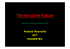### Gravitation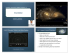### van straten enterprises, inc.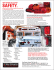### SP-IDL3650s Onebox(EnV161111)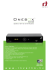### Chapter 5. Gravitation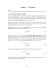### item 9 - Town of Richmond Hill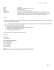### Designing and Building Our Net-Zero Home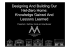### Lectures 1-2: Properties of the Solar System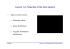### Origin of the Solar System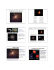### FROM ANTI-GRAVITY TO ZERO-POINT ENERGY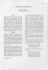### Solar Atlas for the Southern and Eastern - Solar-Med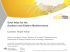### present status and future prospects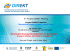### Professor György KOCZISZKY, PhD E-mail: [email protected]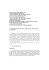### Excellent Triumph GT6 For Sale in Michigan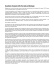### Module P3.2 Gravitation and orbits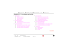### Gravitational lensing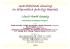### Probing the Dark Matter Density Profile by Galaxy Cluster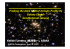### Relativity and Gravitation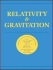### CONTENTS - The Institute of Mathematical Sciences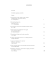### Untitled - Problemi Attuali di Fisica Teorica